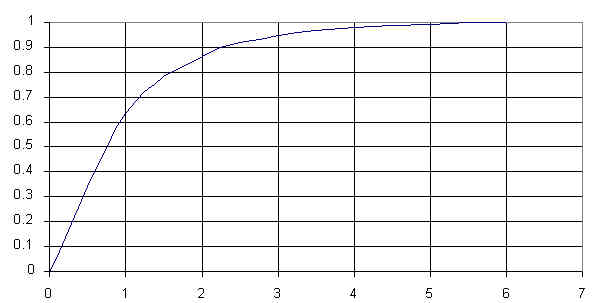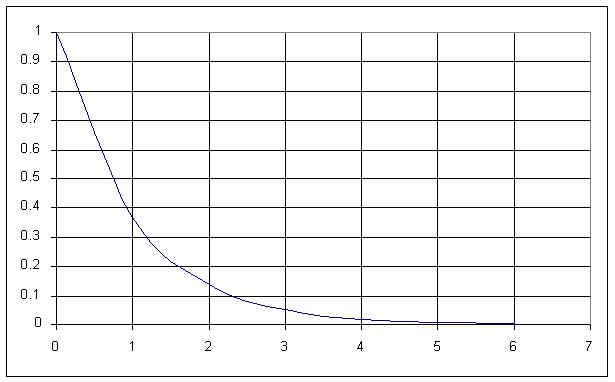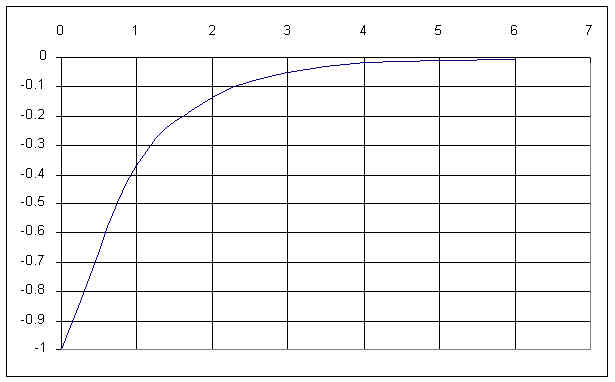Summary of Capacitor and Inductor Facts:

 Property Capacitor Inductor Can't change instantaneously: v i fully charged i=0, v=V  looks like open circuit to DC v=0, i=V/R  looks like short circuit to DC fully discharged v=0, i=V/R  looks like short circuit to DC i=0, v=V  looks like open circuit to DC time constant T=RC T=L/R VOLTAGE       Charging Curve     CURRENT Vfinal=V; Vo=0Io=V/RVo=VIfinal=V/R; Vo=0VOLTAGE           Discharging curve         CURRENT Vo=VIo=-V/RVo=-IRIo=IProperty Capacitor Inductor Basic Formula Q=CV, C=Q/V, V=Q/C  i=C(dv/dt) v=L(di/dt) Energy Stored W=0.5CV^2 W=0.5LI^2 physical C= kA/d L=kAN^2/L Series combination 1/Ct = 1/C1 + 1/C2 + 1/C3 +... Lt=L1 + L2 + L3 +... Parallel combination Ct = C1 + C2 + C3 + ... 1/Lt=1/L1 + 1/L2 + 1/L3 +... Reactance (AC) Xc=1/(2*pi*f*C) Xl=2*pi*f*L Reactive Power: Pr=VrmsIrms Pr=V^2/Xc = I^2 Xc Pr=V^2/Xl = I^2 Xl

Universal Curves:

 Ending at a final value v=V(1-e^(-t/T))starts at zero, ends at positive value number of time constants (T) % of final value 0 0% 1 63% 2 86% 3 95% 4 98% 5 99% (considered 100%) Ending at zero v=Ve^(-t/T)starts at a positive value; ends at zero; v= -Ve^(-t/T)starts at a negative value; ends at zero; number of time constants (T) % of initial value 0 100% 1 37% 2 14% 3 5% 4 2% 5 1% (considered zero)

3/28/02-dw